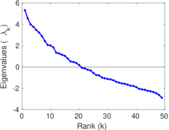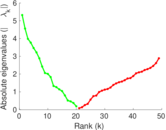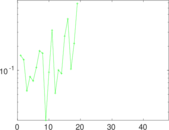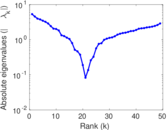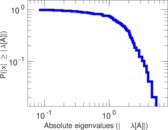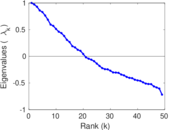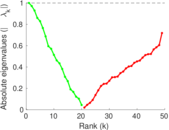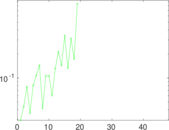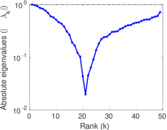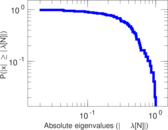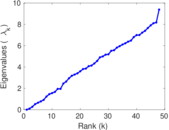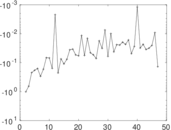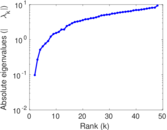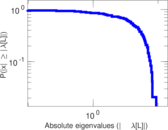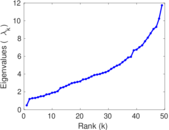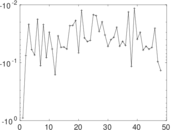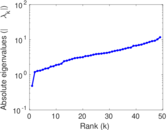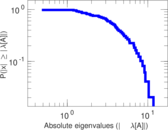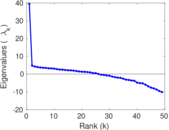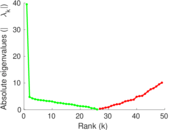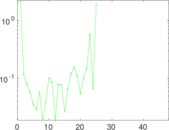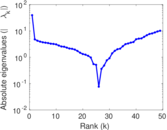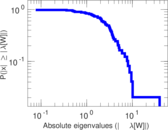# Contiguous USA

These are the 48 contiguous states and the District of Columbia of the United States of America (the USA). They include all states except the states of Alaska and Hawaii, which are not connected by land with the other states, and include the District of Columbia (DC). An edge denotes that two states share a border. The US states in the configuration given by this dataset exist since February 14, 1912, when Arizona was admitted as the 48th state, and is current as of 2014. The states of Alaska and Hawaii were admitted as the 49th and 50th states in 1959, but are not contiguous with the other states, and are not reflected in this dataset.

 Code `CU` Internal name `contiguous-usa` Name Contiguous USA Data source http://www-cs-faculty.stanford.edu/~uno/sgb.html AvailabilityDataset is available for download Consistency checkDataset passed all tests Category Infrastructure network Dataset timestamp 1912 Node meaning State Edge meaning Border Network format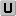Unipartite, undirected Edge typeUnweighted, no multiple edges LoopsDoes not contain loops

## Statistics

 Size n = 49 Volume m = 107 Loop count l = 0 Wedge count s = 421 Claw count z = 494 Cross count x = 382 Triangle count t = 57 Square count q = 70 4-Tour count T4 = 2,458 Maximum degree dmax = 8 Average degree d = 4.367 35 Fill p = 0.090 986 4 Size of LCC N = 49 Diameter δ = 11 50-Percentile effective diameter δ0.5 = 3.476 64 90-Percentile effective diameter δ0.9 = 6.983 76 Median distance δM = 4 Mean distance δm = 4.255 02 Gini coefficient G = 0.201 221 Balanced inequality ratio P = 0.425 234 Relative edge distribution entropy Her = 0.982 602 Power law exponent γ = 1.715 37 Tail power law exponent γt = 8.991 00 Tail power law exponent with p γ3 = 8.991 00 p-value p = 0.668 000 Degree assortativity ρ = +0.233 397 Degree assortativity p-value pρ = 0.000 577 516 Clustering coefficient c = 0.406 176 Spectral norm α = 5.318 55 Algebraic connectivity a = 0.098 048 7 Spectral separation |λ1[A] / λ2[A]| = 1.166 23 Non-bipartivity bA = 0.456 938 Normalized non-bipartivity bN = 0.281 820 Algebraic non-bipartivity χ = 0.483 588 Spectral bipartite frustration bK = 0.027 682 0 Controllability C = 3 Relative controllability Cr = 0.061 224 5

## Plots

### Fruchterman–Reingold graph drawing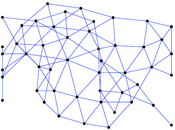### Degree distribution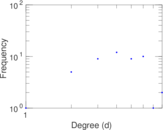### Cumulative degree distribution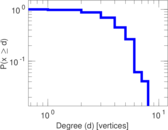### Lorenz curve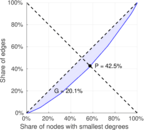### Spectral distribution of the adjacency matrix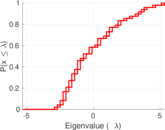### Spectral distribution of the normalized adjacency matrix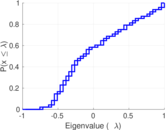### Spectral distribution of the Laplacian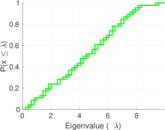### Spectral graph drawing based on the adjacency matrix### Spectral graph drawing based on the Laplacian### Spectral graph drawing based on the normalized adjacency matrix### Degree assortativity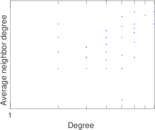### Zipf plot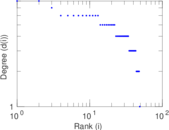### Hop distribution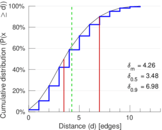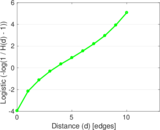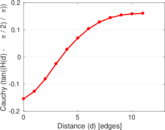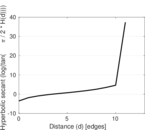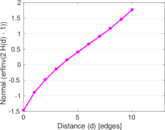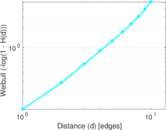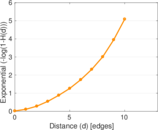### Double Laplacian graph drawing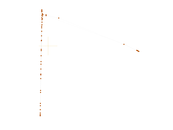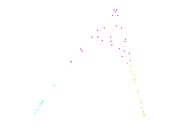### Delaunay graph drawing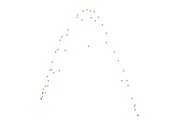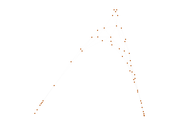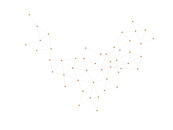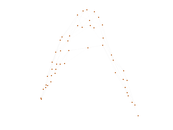### Clustering coefficient distribution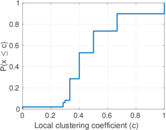### Average neighbor degree distribution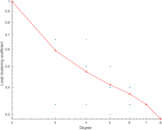### SynGraphy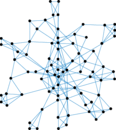### Matrix decompositions plots